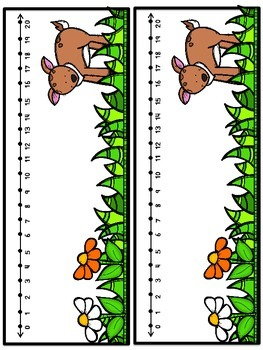# Spring & Summer Interactive Math PrintablesPreK - 1st, Homeschool
Subjects
Standards
Resource Type
Formats Included
• PDF
Pages
78 pages

### Description

So excited about this pack! It includes 30 hands-on, interactive printables that hits on EVERY SINGLE kindergarten math common core standard. I specifically made sure every single standard had a hands-on activity to go with it! These a SO not your average worksheets ;) Each standard has an activity to go with it (sometimes two!)

CCSS.MATH.CONTENT.K.CC.A.1
Count to 100 by ones and by tens.
CCSS.MATH.CONTENT.K.CC.A.2
Count forward beginning from a given number within the known sequence(instead of having to begin at 1).
CCSS.MATH.CONTENT.K.CC.A.3
Write numbers from 0 to 20. Represent a number of objects with a written numeral 0-20 (with 0 representing a count of no objects).
CCSS.MATH.CONTENT.K.CC.B.4
Understand the relationship between numbers and quantities; connect counting to cardinality.
CCSS.MATH.CONTENT.K.CC.B.4.A
When counting objects, say the number names in the standard order, pairing each object with one and only one number name and each number name with one and only one object.
CCSS.MATH.CONTENT.K.CC.B.4.B
Understand that the last number name said tells the number of objects counted. The number of objects is the same regardless of their arrangement or the order in which they were counted.
CCSS.MATH.CONTENT.K.CC.B.4.C
Understand that each successive number name refers to a quantity that is one larger.
CCSS.MATH.CONTENT.K.CC.B.5
Count to answer "how many?" questions about as many as 20 things arranged in a line, a rectangular array, or a circle, or as many as 10 things in a scattered configuration; given a number from 1-20, count out that many objects.
CCSS.MATH.CONTENT.K.CC.C.6
Identify whether the number of objects in one group is greater than, less than, or equal to the number of objects in another group, e.g., by using matching and counting strategies.
CCSS.MATH.CONTENT.K.CC.C.7
Compare two numbers between 1 and 10 presented as written numerals.
CCSS.MATH.CONTENT.K.OA.A.1
Represent addition and subtraction with objects, fingers, mental images, drawings, sounds (e.g., claps), acting out situations, verbal explanations, expressions, or equations.
CCSS.MATH.CONTENT.K.OA.A.2
Solve addition and subtraction word problems, and add and subtract within 10, e.g., by using objects or drawings to represent the problem.
CCSS.MATH.CONTENT.K.OA.A.3
Decompose numbers less than or equal to 10 into pairs in more than one way, e.g., by using objects or drawings, and record each decomposition by a drawing or equation (e.g., 5 = 2 + 3 and 5 = 4 + 1).
CCSS.MATH.CONTENT.K.OA.A.4
For any number from 1 to 9, find the number that makes 10 when added to the given number, e.g., by using objects or drawings, and record the answer with a drawing or equation.
CCSS.MATH.CONTENT.K.OA.A.5
Fluently add and subtract within 5.
CCSS.MATH.CONTENT.K.NBT.A.1
Compose and decompose numbers from 11 to 19 into ten ones and some further ones, e.g., by using objects or drawings, and record each composition or decomposition by a drawing or equation (such as 18 = 10 + 8); understand that these numbers are composed of ten ones and one, two, three, four, five, six, seven, eight, or nine ones.
CCSS.MATH.CONTENT.K.MD.A.1
Describe measurable attributes of objects, such as length or weight. Describe several measurable attributes of a single object.
CCSS.MATH.CONTENT.K.MD.A.2
Directly compare two objects with a measurable attribute in common, to see which object has "more of"/"less of" the attribute, and describe the difference. For example, directly compare the heights of two children and describe one child as taller/shorter.
CCSS.MATH.CONTENT.K.MD.B.3
Classify objects into given categories; count the numbers of objects in each category and sort the categories by count.
CCSS.MATH.CONTENT.K.G.A.1
Describe objects in the environment using names of shapes, and describe the relative positions of these objects using terms such as above, below, beside, in front of, behind, and next to.
CCSS.MATH.CONTENT.K.G.A.2
Correctly name shapes regardless of their orientations or overall size.
CCSS.MATH.CONTENT.K.G.A.3
Identify shapes as two-dimensional (lying in a plane, "flat") or three-dimensional ("solid").
CCSS.MATH.CONTENT.K.G.B.4
Analyze and compare two- and three-dimensional shapes, in different sizes and orientations, using informal language to describe their similarities, differences, parts (e.g., number of sides and vertices/"corners") and other attributes (e.g., having sides of equal length).
CCSS.MATH.CONTENT.K.G.B.5
Model shapes in the world by building shapes from components (e.g., sticks and clay balls) and drawing shapes.
CCSS.MATH.CONTENT.K.G.B.6
Compose simple shapes to form larger shapes. For example, "Can you join these two triangles with full sides touching to make a rectangle?"

Enjoy and HAVE FUN!

Also, please comment, rate for tpt credits :)

~Chelsea {The Twenty Something Teacher}
http://twentysomethingteacher.blogspot.com/
Total Pages
78 pages
N/A
Teaching Duration
N/A
Report this Resource to TpT
Reported resources will be reviewed by our team. Report this resource to let us know if this resource violates TpT’s content guidelines.

### Standards

to see state-specific standards (only available in the US).
Compose simple shapes to form larger shapes. For example, “Can you join these two triangles with full sides touching to make a rectangle?”
Model shapes in the world by building shapes from components (e.g., sticks and clay balls) and drawing shapes.
Analyze and compare two- and three-dimensional shapes, in different sizes and orientations, using informal language to describe their similarities, differences, parts (e.g., number of sides and vertices/“corners”) and other attributes (e.g., having sides of equal length).
Identify shapes as two-dimensional (lying in a plane, “flat”) or three-dimensional (“solid”).
Correctly name shapes regardless of their orientations or overall size.